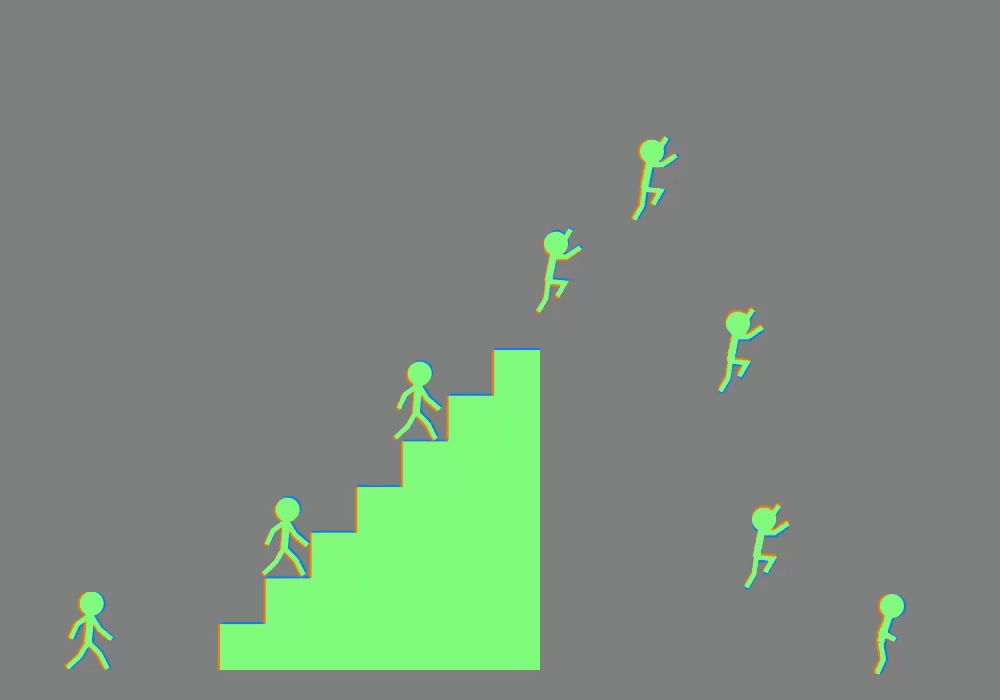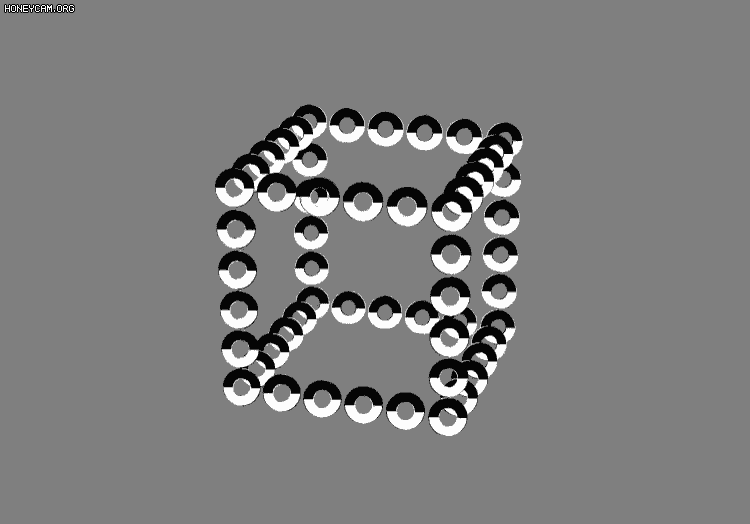# 来看看怎么用OpenCV解构Twitter大牛jagarikin的视觉错觉图

Hello，大家好，我是桔子菌，今天我们来看些有趣的视觉错觉图。Twitter上有个叫jagarikin的大牛发布了很多这种图片。像下面这张图片里，你是不是看到这些小人在爬楼梯，爬完了又往下跳？图源：Twitter@jagarikin图源：Twitter@jagarikin

``````#juzicode.com VX:桔子code
import os
import time
import cv2
def split_image(fn,path='.\\'):
'''
从gif中分离图片
'''
fn_full_path = path+fn
cap = cv2.VideoCapture(fn_full_path)
count_img = 0
while True:
flag,img = cap.read()
if flag is not True:break
cv2.imshow('img',img)
cv2.waitKey(10)
cv2.imwrite(str(count_img)+'.jpg',img)
count_img += 1
print('count_img=',count_img)

if name == "__main__":
img_fn = '1.gif'
split_image(img_fn)``````

``````path = '.\\'
img_files = [f for f in os.listdir(path) if '.jpg' in f]
for  file in img_files:
img_dest = cv2.imread(path+file)
show_img('img_dest VX:juzicode/juzicode.com',img_dest,is_show=dbg_is_show,wait_time=100)``````

``````img_hsv = cv2.cvtColor(img_dest, cv2.COLOR_BGR2HSV)#RGB转换为hsv
show_img("hsv", img_hsv,wait_time=100)
img_h, img_s, img_v = cv2.split(img_hsv) #分离hsv各分量
show_img('img_h',img_h,is_show=dbg_is_show,wait_time=200)
show_img('img_s',img_s,is_show=dbg_is_show,wait_time=100)
show_img('img_v',img_v,is_show=dbg_is_show,wait_time=200)  ``````

``````thresh_bin,img_bin= cv2.threshold(img_s,30,255,cv2.THRESH_BINARY)
print('thresh_bin',thresh_bin )
show_img('img_bin',img_bin,wait_time=100,is_show=dbg_is_show)  ``````

``````res = cv2.findContours(img_bin,cv2.RETR_TREE,cv2.CHAIN_APPROX_SIMPLE)
contours=res
print ('len(contours):',len(contours))
for i in range(0,len(contours)):
x, y, w, h = cv2.boundingRect(contours[i])#提取起点、宽高
print(i,len(contours[i]))
if len(contours[i])<80:continue #去除噪声
text='x,y=(%d,%d) w,h=(%d,%d)'%(x, y, w, h)
if x< 150: #这里只显示左侧圆的内外圆起始位置和宽高
cv2.putText(img_dest, text, (x,y-10 ), cv2.FONT_HERSHEY_SIMPLEX, 0.8, (0, 255, 0), 2)
cv2.rectangle(img_dest, (x,y), (x+w,y+h), (0,0,255), 3)
show_img("img_dest_rect", img_dest,wait_time=100) ``````图源：Twitter@jagarikin